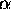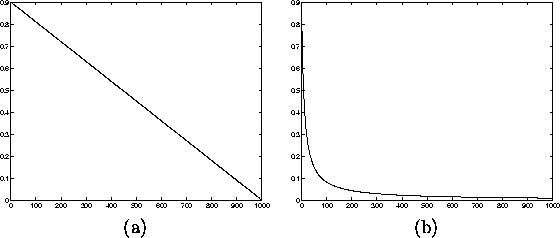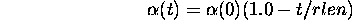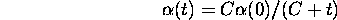Next: Visualization Up: Training Previous: Neighborhood function

### Learning rate

Learning rate is a decreasing function of time. Two forms that are commonly used are a linear function of time and a function that is inversely proportional to the time t. These are illustrated in the Figure 2.7. Linear alpha function (a) decreases to zero linearly during the learning from its initial value whereas the inverse alpha function (b) decreases rapidly from the initial value Both the functions in the Figure have the initial value of 0.9. The initial values formust be determined. Usually, when using a rapidly decreasing inverse alpha function, the initial values can be larger than in the linear case. The learning is usually performed in two phases. On the first round relatively large initial alpha values are used () whereas small initial alpha values () are used during the other round. This corresponds to first tuning the SOM approximately to the same space than the inputs and then fine-tuning the map. There are several rules-of-thumb for picking suitable values. These have been found through experiments and can be found in the monograph by Kohonen .Figure 2.7: Learning rates as functions of time

Alpha values are defined to befor the linear case andfor the inverse function. C can be, for example C = rlen / 100. rlen is the running length of the training, or number of samples fed to the network. These are the values used in the programming package SOM_PAK .

By choosing a suitable initial learning rate and a suitable form for the learning rate function, we can considerably affect the result.

Jaakko Hollmen
Fri Mar 8 13:44:32 EET 1996